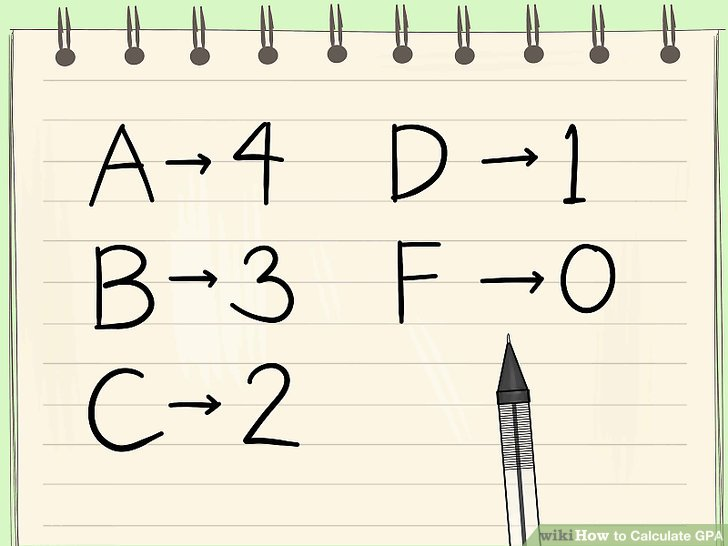# How To Calculate Your GPAWith the first trimester ending, students are all excited for (or dreading) their grades. With this comes the question of GPAs. The GPA is an important tool to determine how well a student is doing academically. Many students, however, have no idea what a GPA is or how it is calculated.

“GPA” is an acronym for “Grade Point Average,” a tool to measure your average grade throughout all classes. The method Northwood uses is very simple, and it’s easy for students to find on their own. It takes the total points from the letter grades and averages them to find what is essentially the average grade. The letter/point correspondence works as seen below:

A   =  4.0
A-  =  3.7
B+ =  3.3
B   =  3.0
B-  =  2.7
C+ =  2.3
C   =  2.0
C-  =  1.7
D+ =  1.3
D   =  1
F   =   0

Northwood does not include A+ or D- in their grading scale. Any grade above a 97 is an A, and any grade below a 60 is an F, while 60 – 66.9 is a D.

In the case of Honors and AP courses, Northwood includes a “weighted” grading system. For an honors level class, 0.5 is added to the grade point, while for an AP class, 1.0 is added. For example, a B for a normal level class is 3.0, an honors class is 3.5, and an AP class is 4.0. Let’s take this hypothetical grade sheet:

 Spanish III Honors Physics English 11 AP US History Pre-Calculus Guitar A B A- C+ B- B+

To find the GPA, first convert them into their point values:

Spanish = 4.0
Honors physics = 3.0 + .5
English 11 = 3.7
AP US History = 2.3 + 1.0
Pre-Calculus = 2.7
Guitar = 3.3
Then add them together: 4.0 + 3.5 + 3.7 + 3.3 + 2.7 + 3.3 = 20.5
And divide by the number of classes (6): 20.5 / 6 = 3.416

Thus, the Grade Point Average is 3.416, or a high B+.

Not every school uses this exact method for the GPA. Some schools do not include art courses in the GPA calculation, and some do not count AP and Honors classes any higher than normal level classes. This, however, is how Northwood’s works, and now hopefully you can calculate it on your own.

The Mirror was established in 1927
© 2015-2019 by the Staff of The Mirror
The Mirror's Policy Manual and Style Guide.
The Mirror is funded by gifts to the Northwood Fund. Thank you.